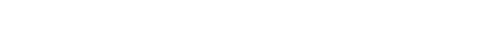glDepthFunc.3glOpenGL man pages

```

```

## Name

```  glDepthFunc -	specify	the value used for depth buffer	comparisons

```

## C Specification

```  void glDepthFunc( GLenum func	)

```

## Parameters

```
func	Specifies the depth comparison function.  Symbolic constants
GL_NEVER, GL_LESS, GL_EQUAL, GL_LEQUAL,	GL_GREATER, GL_NOTEQUAL,
GL_GEQUAL, and GL_ALWAYS are accepted.	The default value is GL_LESS.

```

## Description

```  glDepthFunc specifies	the function used to compare each incoming pixel z
value	with the z value present in the	depth buffer.  The comparison is
performed only if depth testing is enabled.  (See glEnable and glDisable of
GL_DEPTH_TEST.)

func specifies the conditions	under which the	pixel will be drawn.  The
comparison functions are as follows:

GL_NEVER	      Never passes.

GL_LESS	      Passes if	the incoming z value is	less than the stored
z	value.

GL_EQUAL	      Passes if	the incoming z value is	equal to the stored z
value.

GL_LEQUAL	      Passes if	the incoming z value is	less than or equal to
the stored z value.

GL_GREATER	      Passes if	the incoming z value is	greater	than the
stored z value.

GL_NOTEQUAL	      Passes if	the incoming z value is	not equal to the
stored z value.

GL_GEQUAL	      Passes if	the incoming z value is	greater	than or	equal
to the stored z value.

GL_ALWAYS	      Always passes.

The default value of func is GL_LESS.	 Initially, depth testing is
disabled.

```

## Errors

```  GL_INVALID_ENUM is generated if func is not an accepted value.

GL_INVALID_OPERATION is generated if glDepthFunc is executed between the
execution of glBegin and the corresponding execution of glEnd.

```

## Associated Gets

```  glGet	with argument GL_DEPTH_FUNC
glIsEnabled with argument GL_DEPTH_TEST

```

```  glDepthRange,	glEnable